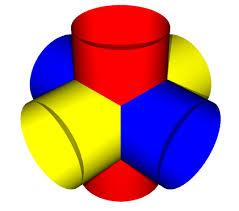# Calculate 3016

Calculate the height of the cylinder if you know its surface S and the radius of the base r.
a) r = 2 cm, S = 120 cm square
b) r = 7 dm, S = 4,000 dm square
c) r = 0.2 m, S = 20 square meters

a =  7.5493 cm
b =  83.9457 dm
c =  15.7155 m

### Step-by-step explanation:Did you find an error or inaccuracy? Feel free to write us. Thank you!